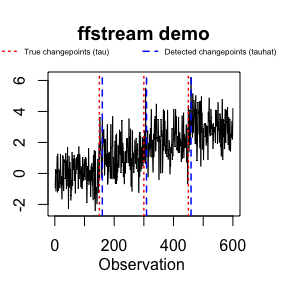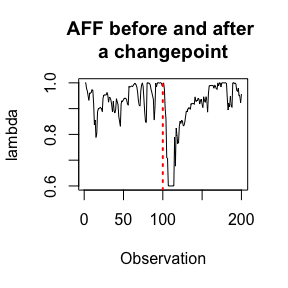# Introduction

The ffstream package provides an implementation for the Adaptive Forgetting Factor (AFF) change detector (Bodenham and Adams, 2016). In fact, the following four online change detection methods are implemented:

• AFF (Bodenham and Adams, 2016)
• FFF (Bodenham and Adams, 2016)
• CUSUM (Page, 1954)
• EWMA (Roberts, 1959)

While both AFF and FFF were proposed recently, CUSUM and EWMA are classical sequential change detectors from the 1950s. However, since I could not find implementations of CUSUM and EWMA on CRAN, I decided to include them in the ffstream package (they are used as baseline comparison methods in the paper mentioned above, which contains detailed descriptions of all four methods).

## Demo

If you want to quickly see the AFF method in action, there is a function demo_ffstream() which provides a (good) example* of how AFF can perform:

(*many streams will have changepoints that are more difficult to detect!)

library(ffstream)
result <- demo_ffstream(showPlot=TRUE, plotSmall=TRUE) #'plotSmall' only needed for vignetteHere, the true changepoints, labelled tau, are shown as the vertical red dotted lines, while the detected (estimated) changepoints, labelled tauhat, are shown as blue dashed lines. Note that the showPlot=TRUE flag is optional. The values are contained in the result list:

print(result)
## $tau ##  150 300 450 ## ##$tauhat
##  160 309 459
##
## $method ##  "AFF" ## ##$param
##   alpha   eta BL
## 1  0.01 0.001 50

It is also possible to return the vector of observations as part of the result list by using the returnStream flag:

result <- demo_ffstream(returnStream=TRUE)

## Quick start

Here is a quick example of how to use the AFF method to detect a change in a vector of observations:

#a simple stream with changepoints at tau=100, 200, 300
set.seed(5)
x <- rnorm(400, 0, 1) + rep(c(0:3), each=100)

#use the AFF method to detect changepoints in this stream
library(ffstream)
result <- detectAFFMean(x)
print(result)
## $tauhat ##  113 206 303 382 In this example, • the first three changepoints tauhat=113, 206, 303 could be considered correct detections, • but the last value, tauhat=382 is a false detection, since there is no changepoint after 300. The rest of the vignette describes the many different options available for the change detectors: multiple or single changes, using known prechange means and variances, sequential or batch processing, etc. ## Implementation The implementation in R uses the (Rcpp package) to allow the core code to be written in C++. There are two ways to call the change detectors implemented here: • initialise and sequentially update • detect a change in a vector of observations (but still process observations sequentially) # Initialise and sequentially update This is the scenario for which the methods were designed; monitoring a data stream of observations, and as each observation arrives, the detector is (sequentially) updated. ## Initialisation Initialising a change detector object is very easy. Here are four examples below: library(ffstream) # initialise an AFF change detector with alpha=0.01, eta=0.001 and burn-in length 30 affcd <- initAFFMeanCD(alpha=0.01, eta=0.001, BL=30) # initialise a FFF change detector with lambda=0.99, alpha=0.05, and burn-in length 50 fffcd <- initFFFMeanCD(lambda=0.99, alpha=0.05, BL=50) # initialise a CUSUM change detector with h=0.50, k=4.77, and burn-in length 70 cusumcd <- initCUSUMMeanCD(h=0.50, k=4.77, BL=70) # initialise a EWMA change detector with r=0.25, L=3.023, and burn-in length 50 ewmacd <- initEWMAMeanCD(r=0.25, L=3.023, BL=50) ## Updating sequentially Updating is also easy (the syntax is the same for all detectors, so only the AFF method is shown): #generate an observation x <- rnorm(1, mean=0, sd=1) #update the detector affcd$update(x)

#generate another observation
x <- rnorm(1, mean=0, sd=1)

#update the detector
affcd$update(x) #etc... ### Sequentially processing a vector Alternatively, one can sequentially process a vector using a for-loop: #generate a simple stream - same as in the 'Quick start' example above set.seed(5) x <- rnorm(400, 0, 1) + rep(c(0:3), each=100) #initialise a new aff change detector object affcd2 <- initAFFMeanCD(alpha=0.01, eta=0.01, BL=50) index <- 0 for (obs in x){ affcd2$update(obs)

index <- index + 1
if (affcd2$changeDetected) cat("change detected at observation: ", index, "\n", sep="") } ## change detected at observation: 113 ## change detected at observation: 206 ## change detected at observation: 303 ## change detected at observation: 382 The changepoints detected (tauhat) in this example are the same as in the Quick start example above, because the same stream was used, and the AFF was initialised with the same parameters (alpha=0.01 and eta=0.01 are the default values). The example shows a field that each change detector has: • changeDetected is a boolean which specifies if a change has just been detected after the most recent update. • After detecting a change, in a multiple change detection scenario the detector will immediately switch to a burn-in state where it estimates the mean and variance of the stream. # Detecting changes in vectors As the example in the Quick start section shows, instead of initialising a detector object and sequentially updating as in the section above, it is also possible to process a vector directly. The following four methods are available: • detectAFFMean • detectFFFMean • detectCUSUMMean • detectEWMAMean It is important to note that each method requires different control parameters, as shown in the examples below. ## Three modes of detection There are also three modes for each detector: • detect multiple changepoints, using a burn-in period to estimate the mean and variance for each new regime, • detect a single changepoint, using a burn-in period to estimate the mean and variance for the first regime, • detect a single changepoint, but use the known prechange mean and variance values (if known). Althought the multiple changepoint case is perhaps the most interesting, the single changepoint option is available, since it is the case most often considered in the literature. ## Examples #generate a simple stream - same as in the 'Quick start' example above set.seed(8) x <- rnorm(400, 5, 1) + rep(c(0:3), each=100) # mean is 5 and s.d. is 1 #multiple changepoints list_aff <- detectAFFMean(x, alpha=0.01, eta=0.01, BL=50, multiple=TRUE) #now only a single (the first) changepoint list_aff2 <- detectAFFMean(x, alpha=0.01, eta=0.01, BL=50, single=TRUE) #now only a single (the first) changepoint, but with the prechange mean and variance known list_aff3 <- detectAFFMean(x, alpha=0.01, eta=0.01, single=TRUE, prechangeMean=5, prechangeSigma=1) #similar for FFF, CUSUM and EWMA: list_fff <- detectFFFMean(x, alpha=0.01, lambda=0.95, BL=50, multiple=TRUE) list_cusum <- detectCUSUMMean(x, k=0.25, h=8.00, BL=50, multiple=TRUE) list_ewma <- detectEWMAMean(x, r=0.25, L=3.023, BL=50, multiple=TRUE) resultsList <- list("aff"=list_aff$tauhat, "fff"=list_fff$tauhat, "cusum"=list_cusum$tauhat, "ewma"=list_ewma$tauhat, "aff_single"=list_aff2$tauhat, "aff_single_prechange"=list_aff3$tauhat) print(resultsList) ##$aff
##  117 212 313
##
## $fff ##  116 212 313 ## ##$cusum
##  115 213 310
##
## $ewma ##  115 307 ## ##$aff_single
##  117
##
## $aff_single_prechange ##  117 This example shows that, for this simple case, the detectors can have very similar detection performance. # Further details: change detectors This section provides a bit more information on how to use the change detector objects. Further information can be found by looking at the R documentation. ## Initialisation, Methods and fields When initialising a change detector using either: • initAFFMeanCD • initFFFMeanCD • initCUSUMMeanCD • initEWMAMeanCD then the following methods and are available to all: • update: update the detector with a single observation, • processVectorSave: batch process a vector of observations and return a list with the field tauhat, the estimated changepoints in that vector, • print: print the current state of the detector. The following fields are then available: • changeDetected: a boolean specifying whether or not a change has been detected after the most recent observation, • streamEstMean: the estimated mean of the stream; if a prechange value is known, it can be set (see examples below), • streamEstSigma: the estimated standard deviation of the stream; if a prechange value is known (see examples below). In practice, the most important method is update, and the most important field is changeDetected. ## Examples #generate a simple stream - same as in the 'Quick start' example above set.seed(5) x <- rnorm(400, 5, 3) + rep(c(0:3), each=100) # mean is 5 and s.d. is 3 #initialise a new aff change detector object affcd3 <- initAFFMeanCD(alpha=0.01, eta=0.01, BL=50) affcd3$streamEstMean <- 5
affcd3$streamEstSigma<- 3 #list containing tauhat, the estimated changepoints returnList <- affcd3$processVectorSave(x)

# Adaptive estimation of the mean

Along with the four change detectors above, it is also possible to only compute the AFF and FFF means (i.e. no change detection).

## Initialisation

The two initialisation functions are which can be used to compute the AFF mean or the FFF mean.:

• initAFFMean which allows one to set the value of eta (default value 0.01),
• initFFFMean which allows one set the value of lambda (default value 1, i.e. no forgetting),

See (Bodenham and Adams, 2016) for further details.

## Updating

The important methods in this case are:

• update: to sequentially update the mean,
• processVector: to batch update the mean by passing a vector,
• processVectorSave: (only for AFF) to batch update the mean AND return a vector of the adaptive forgetting factor $$\overrightarrow{\lambda}$$.

## Examples

#initialise an AFF mean estimator
aff <- initAFFMean(eta=0.01) #it is not necessary to specify eta

#update sequentially
obs <- rnorm(1)
aff$update(obs) #update with a vector vec <- rnorm(20) aff$processVector(vec)

#in order to get the value of the aff back:
aff <- initAFFMean(eta=0.01) #reset first
thelist <- aff$processVectorSave(vec) lambda <- thelist$lambda

#initialise an FFF mean estimator
fff <- initFFFMean(lambda=0.95) #specify lambda, otherwise default is lambda=1, no forgetting
fff$processVector(vec) Here is another example showing the behaviour of the AFF before/after a changepoint: aff <- initAFFMean(eta=0.01) #create a stream set.seed(7) x <- rnorm(200) + rep(c(0, 2), each=100) #lambda thelist <- aff$processVectorSave(x)

titlestr <- "AFF before and after \na changepoint"
plot(thelist\$lambda, type='l', col="black", xlab="Observation", ylab="lambda", main=titlestr)
abline(v=100, col="red", lty="dotted", lwd=2)As the figure shows, the AFF is usually close to 1 (well, in the range [0.8, 1]) before the changepoint at tau=100, then drops dramatically — note that it is truncated at 0.6, for reasons explained in the paper — and then after a period of stability, it increases again. This is the typical, and desired, behaviour of the AFF.